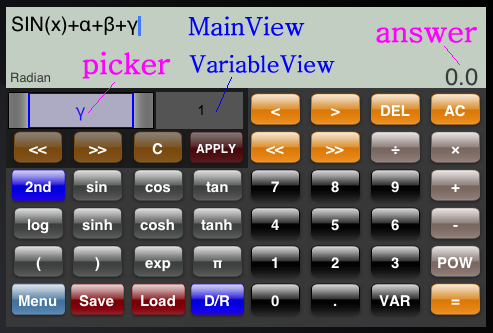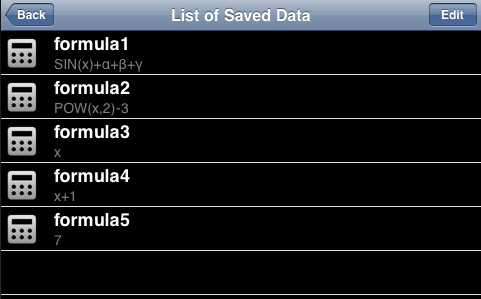# Calc## Buttons For Input

• 0-9
• Use these buttons to input number.

• (
• Use this button to input "(".

• )
• Use this button to input ")".

• +,-,÷,×,=
• Use these buttons to input operator.
Note
「×」 is displayed as "*".
「÷」 is displayed as "/".

• POW
• POW(x, y)
This function returns x raised to the power of y.

• sin
• sin(x)
Returns the sine of a radian angle x in the radian mode. And returns the sine of a degree angle x in the degree mode.

• cos
• cos(x)
Returns the cosine of a radian angle x in the radian mode. And returns the cosine of a degree angle x in the degree mode.

• tan
• tan(x)
Returns the tangent of a radian angle x in the radian mode. And returns the tangent of a degree angle x in the degree mode.

• asin
• sin(x)
Returns the arc sine of a radian angle x in the radian mode. And returns the arc sine of a degree angle x in the degree mode.

• acos
• cos(x)
Returns the arc cosine of a radian angle x in the radian mode. And returns the arc cosine of a degree angle x in the degree mode.

• atan
• tan(x)
Returns the arc tangent of a radian angle x in the radian mode. And returns the arc tangent of a degree angle x in the degree mode.

• sinh
• sinh(x)
Returns the hyperbolic sine of x. If in the degree mode, x is converted automatically as follows.
x = x * π/180.

• cosh
• cosh(x)
Returns the hyperbolic cosine of x. If in the degree mode, x is converted automatically as follows.
x = x * π/180.

• tanh
• tanh(x)
Returns the hyperbolic tangent of x. If in the degree mode, x is converted automatically as follows.
x = x * π/180.

• asinh
• sinh(x)
Returns the arc hyperbolic sine of x. If in the degree mode, retuned value is converted automatically as follows.
RetunedValue = 180 * RetunedValue/π

• acosh
• cosh(x)
Returns the arc hyperbolic cosine of x. If in the degree mode, retuned value is converted automatically as follows.
RetunedValue = 180 * RetunedValue/π

• atanh
• tanh(x)
Returns the arc hyperbolic tangent of x. If in the degree mode, retuned value is converted automatically as follows.
RetunedValue = 180 * RetunedValue/π

• log
• log(x)
This function returns the common logarithm (base-10 logarithm) of x.

• ln
• ln(x)
This function returns returns the natural logarithm (base-e logarithm) of x.

• exp
• EXP(x)
This function returns the value of e raised to the xth power.

• VAR
• Use this button to input the variable which is selected at picker.

• π
• Use this button to input π（=3.141592654）.
Note
This is displayed as "PI".

## Other Buttons

• Use this button to back to the main menu.

• Save
• Use this button to save current formula.

• Use this button to load saved formula.
Note
You can delete saved formula from "Edit" button as follows.• D/R
• This button switches the mode which is radian or degree. The argument of the trigonometric function and the return value relate to this mode. And the argument of the hyperbolic function and the return value relate to this mode too.

• AC
• Use this button to clear formula in the MainView.

• DEL
• Use this button to delete one character at caret.

• 2nd
• Use this button to switch function buttons.

• <<
• In the MainView, caret is moved to the head. In the VariableView, current page of picker is moved to the head.

• >>
• In the MainView, caret is moved to the end. In the VariableView, current page of picker is moved to the end.

• <
• Use this button to move the caret to forward.

• >
• Use this button to move the caret to backward.

• C
• Use this button to clear the value of VariableView.

• APPLY
• Use this button to apply the value to the selected variable at the picker.

## How to use variables

You can use alphabet(a-z) and greek alphabet(α-ω) as the variable in this version.
• You must tap VariableView to start editing the variable, then these zone is enabled.
• If you want to set the value to the variable, You must push the APPLY button after selecting the variable from the picker, and setting the value in the VariableView.
• You must tap MainView to end editing the variable, then these zone is disabled.
• To input the variable on the MainView, push VAR button.